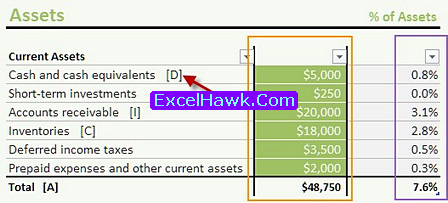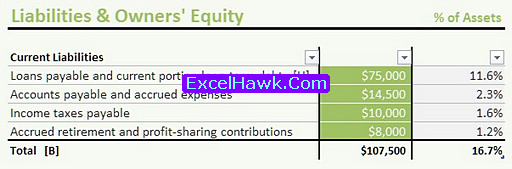# Balance Sheet in Excel Template with Formulas

The balance sheet in excel template served to help in writing and managing the accounting balance sheet. The template mainly purposed for small business company, however the template can used for personal balance sheet excel. This balance sheet format is in ms excel provided with formulas to help in calculating the balance sheet accounts.The balance sheet excel template only has one sheet. In the header (previewed in picture below), are for statistic and ratio. To getting started, first change the temporary name (Contoso, ltd) with the real company name. Then change the period in following field. Both fields are marked with red rectangles.You may realize that the ratio name followed by balance sheet excel format formula, one of the example marked with the red circle in the picture above. If you want to know the formula variable, take a look with Asset and Liabilities & Equity tables. The accounts for the formula variable are marked, example with red arrow in below picture, where the 'A' is actually the Total of Current Assets. The 'B' variable is the Total of Current Liabilities. So, Current Ratio means the Total of Current Assets per Total of Current Liabilities.ASSETS
The assets in balance sheet in excel consist of three categories: Current Asset, Fixed Assets, and Other Assets. You can add new accounts after each category. After the stated amount (in the orange rectangle), the balance sheet analysis excel will automatically calculate the asset percentage (showed in purple rectangle). The percentage based on the assets account per the total of assets (including all the accounts in all categories).

LIABILITIES
The liabilities consist of Current Liabilities and Other Liabilities. You can change or add the liability categories into more specific such as Long Term Liabilities and Short Term one. Like the assets, the percentage comes from the account per the Total of Liabilities and Equities.However, the balance sheet in excel is not complete yet, since there is no equity section. Please don't be worry, it is not too hard to make a new section. You only have to make a category name Equity in the new field. Then add the equity accounts below the category name like the common stock or share. In the Total Owner Equity, replace the previous formula into summary formula that calculate all the equity accounts. Don't forget to change the ratio calculation if it really needed. You have to make sure that summary Total Equity and Total Liabilities equals with Total Asset, or else there must be error in calculations.

SUMMARY
If you want to add new categories into balance sheet in excel, just follow the steps to create Equity accounts. Additionally, it is possible if you want to make charts based on the ratio in the header, or based on accounts or the categories. The balance sheet in excel is served as report template too, so you can automatically print the template with the value and result. The debit credit balance sheet excel can be downloaded at excelhawk.com. Anyway, feel free to edit or modify the balance sheet in excel.